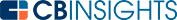# What is the Terminal Value Formula?Terminal value refers to a business’s or asset’s value beyond the forecasting period in which it’s possible to estimate future cash flows.

The forecast period and terminal value are two components of the discounted cash flow (DCF) model. There are two terminal value formulas that you can use to determine the value of a business beyond the forecast period.

The discounted cash flow model is one of the most common models used for business valuation. The DCF model is based on the principle that the value of a business is the present value of all future cash flows. To determine the present value of future cash flows, you use a discount rate.

To determine the future cash flows, you must estimate the future growth rate. Estimating the future growth rate becomes more and more difficult as the time number of years increases. Due to this problem, a terminal value is assigned for cash flows or other financial metrics used.

## How to calculate terminal value

The most common forecast period used for a DCF model ranges from 5-10 years. It’s virtually impossible to estimate the growth rate for a business beyond this period. Beyond the forecast period, you can use a terminal value to indicate all future cash flows beyond the final year for which you can estimate the growth rate. Terminal value can be attached in two ways, explained below.

## Exit multiple

The model used in this method is slightly different from the commonly used method of discounting cash flows. Here, the business value is assumed to be a multiple of a particular metric. The most commonly used metrics are EBITDA, net income, free cash flow, etc. Using the estimated growth rate, calculate the future rate of the selected metric. Then, calculate the terminal value for the final period. In this model, the terminal value will be:

Terminal value (TV) = expected exit multiple in final time period x projected metric

## Perpetuity growth model

In this model, the business is assumed to grow at the same rate forever after the forecast period. This is called the perpetual growth rate. However, use caution because if you use too large a rate, the value will be bloated. If you use an extremely low value, it might lead to undervaluation. Calculate the terminal free cash flow (FCF) as a single term using the perpetual growth rate and discount rate.In this equation, FCF is the free cash flow estimated for the last forecast period, d is the discount rate, and g is the perpetual growth rate. To determine the value of the business, discount the terminal value and other FCFs to the present value.

A terminal value formula is a calculation used in business valuation. There are two terminal value formulas: the perpetuity growth model and the exit multiple method. You can use either formula in the DCF model for business valuation to overcome the challenges of estimating future cash flows beyond the forecasting period

You may also like# Corporate InnovationCorporate StrategyCorporate Venture CapitalVenture CapitalLove Notes